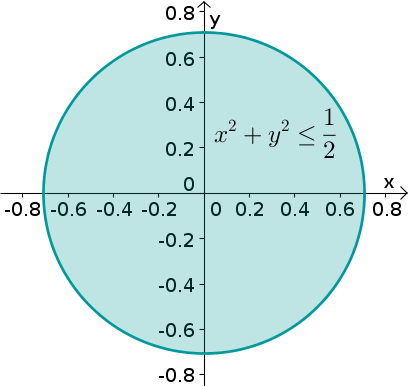# Math Insight

### Image: A disk of radius square root of one halfA disk centered at the origin with radius that is the square root of one half. The equation for the disk is $x^2+y^2 \le \frac{1}{2}$.